Courses
Courses for Kids
Free study material
Free LIVE classes
More

# Photoelectric Effect - Important Concepts for JEE## A Brief Introduction about the Photoelectric Effect

Last updated date: 14th Mar 2023
Total views: 75.3k
Views today: 0.55k

The photoelectric effect is defined as the emission of electrons after a beam of light strikes a metal. Electrons emitted due to the photoelectric effect are called photoelectrons. Albert Einstein stated that any electromagnetic radiation, such as light, is not a wave transmitting through space and is instead a surge of discrete energy particles called photons. The main principle of the photoelectric effect is the law of conservation of energy.

Photoemission is a procedure through which the photoelectrons are emitted, as the light hits the exposed object. The photoelectric effect occurs because the electrons on the material’s surface absorb energy from the incoming light and utilise it to break certain forces that connect them to the nuclei. This effect was primarily put forward by Wilhelm Ludwig Franz Hallwachs in 1887, wherein he noticed that when an incident light falls on the surface of a material at a greater threshold frequency, the radiations are taken inside and electrons are discharged. J.J. Thomson developed the study of cathode rays using the photoelectric effect experiment apparatus in 1889.

## Types of Emission

We’ll be seeing in the coming sections that the emission of electrons from the surface of the metal is only possible if a certain amount of energy is provided to the metal. This energy is equal to what we call the work function of the metal. Now, the energy can be supplied in any form which includes heat energy, light energy, etc. The electron emission has been divided into three types depending on the source of energy used for emission. These types are listed below.

1. Thermionic Emission

As can be guessed from the name of this emission, the energy is supplied in the form of heat. The metal is heated to a sufficient temperature and this provides the energy for the electron emission. When the supplied energy is more than the work function of the metal, electrons are emitted.

1. Field Emission

Field emission is the type of emission that happens due to an electric field. This electric field has to be very strong in order to pull an electron out of the surface of the metal. This is a quantum mechanical process, and in this process, the electrons are able to tunnel through the surface potential barrier.

1. Photoelectric Emission

In photoelectric emission, the source of the energy supply is light. Light of a certain frequency is made to fall on the surface of the metal and if the energy of light is more than the work function of the metal surface, electrons are emitted. We’ll be studying this emission in detail in this article. This kind of emission is also termed as the photoelectric effect.

## List of Formulae Related to the Photoelectric Effect

 S.No. Concept Formula 1. The Planck’s equation $E=h\nu =\dfrac{hc}{\lambda }$ 2. Threshold energy or work function equation $\phi =h{{\nu }_{th}}=\dfrac{hc}{{{\lambda }_{th}}}$ 3. Photon energy and kinetic energy relation ${{E}_{photon}}=\phi +{{E}_{electron}}$ 4. Photoelectric effect equation $h\nu =W+E$ 5. Photon energy equation $E=p\times c$

## Properties of Photon and Law of Photoelectric Emission

We will now look into some of the important properties of photons and discuss the law of photoelectric effect.

Properties of Photons

• All the quantum numbers are zero for a photon.

• Photon is devoid of any mass, electric charge, or part of any electric/magnetic field.

• In an empty space, a photon advances at the speed of light.

• Photons are a type of virtual particles. With an increase or decrease in the photon energy, the frequency also increases and decreases, whereas the wavelength will decrease or increase, respectively.

• The energy of the photons may be expressed as:

$E=p\times c$

where

p = magnitude of the momentum and c = speed of light.

Law of Photoelectric Emission

There are a certain set of laws for successful observation of photoelectric effect or photoelectric emission as mentioned below:

• Time difference between the incident of light and emission of photoelectrons is zero or nil.

• Kinetic energy of the photoelectron is determined by the frequency of incident light.

• Number of photoelectrons emitted is proportional to the intensity of incident light.

• Photoelectrons are ejected only above a certain frequency called cut-off or threshold frequency.

The Planck’s Equation

Albert Einstein proposed Planck's equation in which different light frequencies hold different energies of photons. We can devise from the below equation that the wavelength of light is inversely proportional to the frequency of light. For instance, blue light’s frequency is greater and wavelength is smaller as compared to red light where the frequency is much smaller and wavelength is larger. Also, photon energy for blue light is larger than that of the red light.

$E=h\nu =\dfrac{hc}{\lambda }$

where

E = Photon energy( J), h = Planck’s constant $\left( 6.626\,\times \,{{10}^{-34}}\,\dfrac{J}{s} \right)$

$\nu =$Frequency of light( Hz), c= Speed of light in vacuum $\left( \dfrac{m}{s} \right)$

$\lambda =$ Wavelength of light (nm)

## Types of Photoelectric Effect

We have learned what photoelectric effect is and also discussed some important formulas related to it. Let's now take a brief look at the types of the photoelectric effect.

The photoelectric effect is basically divided into two types:

1. Internal Photoelectric Effect

The discharge of electrons within the object when photons strike the surface of the metal is known as an internal photoelectric effect.

The collection of all the energy levels of the valence electrons is termed as a valence band. When photons make contact with the metal surface, some of the valence electrons which are lower energy level electrons obtain an ample amount of energy and are unbound from the parent atom. They further transmit into the higher energy level conduction band, where the electrons move freely from one region to another.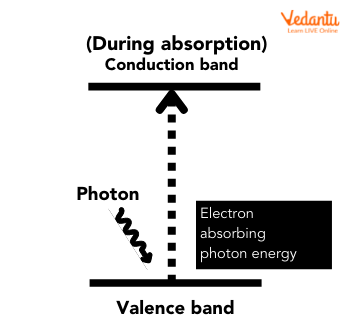Internal Photoelectric Effect

This emission of photoelectrons inside the material is termed internal photoemission or internal photoelectric effect. The internal photoelectric effect is also called the photoconductive effect or photovoltaic effect. The photovoltaic effect is the principle utilised behind the working of solar panels.

1. External Photoelectric Effect

The discharge of electrons from the surface of the metal, when photons strike the metal surface, is known as the external photoelectric effect.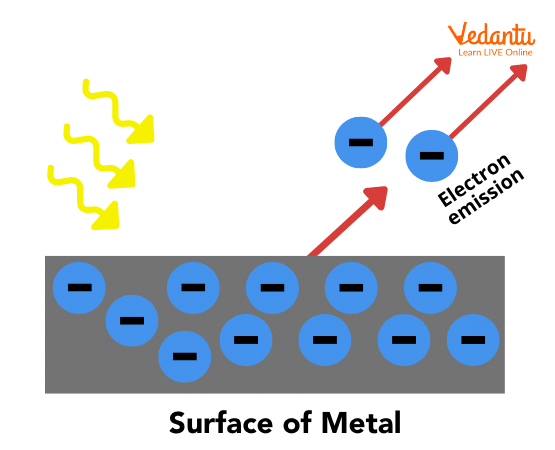External Photoelectric Effect

As shown in the above image, when the light hits the surface of the metal, certain valence electrons and free electrons acquire enough energy to detach from the bonding with the metal and are emitted from the metal surface. The external photoelectric effect happens when the frequency of the incident light is larger than the work function or threshold energy of a metal.

## Explanation of Important Equations of Photoelectric Effect

1. Photoelectric Effect Formula

When the energy of the photon is equivalent to the energy and kinetic energy required to eradicate an electron, it is known as the photoelectric effect equation. It is expressed as follows:

$h\nu =W+E$

where

h= Planck’s constant

$\nu =$Frequency of the incident photon

W= Work function

E= Maximum kinetic energy of ejected electrons $\left( \dfrac{1}{2}m{{v}^{2}} \right)$

2. Threshold Energy for the Photoelectric Effect

Threshold energy $\left( \phi \right)$ is the least amount of energy needed to eliminate an electron from the metallic object.

Threshold Frequency: Threshold frequency is the minimum frequency for which electron emission is possible in the photoelectric effect. If we plot a graph between energy and frequency of the electron, it would be as shown below: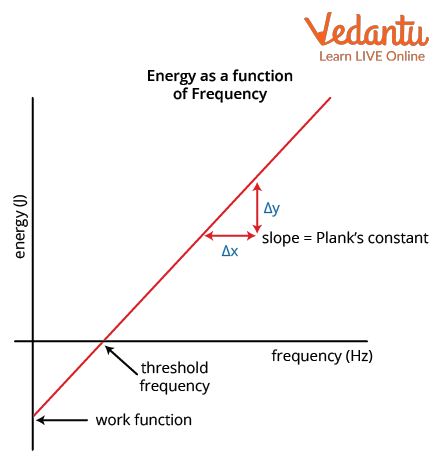Energy-Frequency Graph for Photoelectric Effect

In the graph, we can see that the negative value or intercept of the energy-frequency graph gives the value of work function since work function is the amount of energy on an electron that is required to break it from the metal. At threshold frequency, energy will be zero and the slope of the graph will give the value of Planck's constant.

The relation between threshold energy and threshold frequency  can be given as:

$\phi =h{{\nu }_{th}}=\dfrac{hc}{{{\lambda }_{th}}}$

where

$\phi =$ Threshold energy (J)

h= Planck’s constant $\left( 6.626\,\times \,{{10}^{-34}}\, \dfrac{J}{s} \right)$

${{\nu }_{th}}=$ Threshold frequency $\left( Hz \right)$, $c=$ Speed of light $\left( \dfrac{m}{s} \right)$

${{\lambda }_{th}}=$ Threshold wavelength $\left( nm \right)$

3. Relation Between the Photon Energy and the Kinetic Energy of the Ejected Photoelectron

In the photoelectric effect for the emission of electrons, the energy of the incident photon should be greater than the threshold energy of the metal surface, and the extra energy above the threshold energy should provide the kinetic energy to emitted electrons. Therefore, energy of the incident photon can  be expressed as:

${{E}_{photon}}=\phi +{{E}_{electron}}$

$h \nu=h v_{t h}+\dfrac{1}{2} m_{e} v^{2}$

We can also write

$\dfrac{1}{2} m_{e} v^{2}=h \nu-h \nu_{t h}$

where

Ephoton = Incident photon’s energy

Ephoton = hν

$\phi$ =Threshold energy of the metal surface

$\phi$ = th

Eelectron = Kinetic energy of the photoelectron

Eelectron= $\dfrac{1}{2} m_{e} v^{2}$

${{m}_{e}}=$Mass of electron $(9.1\times {{10}^{-31}}kg)$

If we were to plot a relation between photon energy and kinetic energy of the ejected photoelectron, we could take the kinetic energy of the photoelectron on the y axis and the frequency of the photon on the x axis. The graph that will form between these parameters will be as given below.

The graph shows the linear relationship between the electron kinetic energy and the frequency of the incident photons. This frequency basically represents the energy of the incident photons because when the frequency is multiplied by the Planck's constant, we have the energy.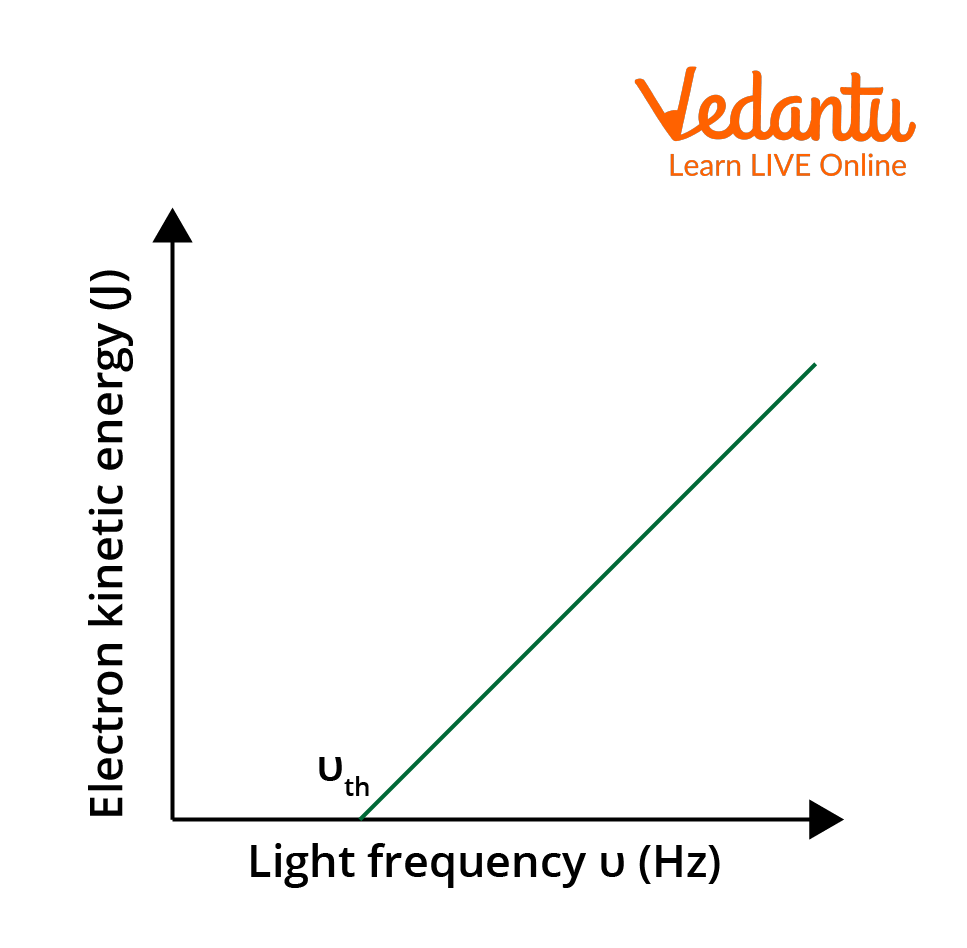Graph for the relation between photon frequency and ejected electron kinetic energy

## Experimental Set Up for Photoelectric Effect

Experimental set up of the photoelectric effect consists of an evacuated tube with an emitter and collector. An emitter is a photosensitive material which emits electrons when monochromatic light entering from a quartz window falls upon it. The collector receives the emitted electrons from the emitter and the flow of electrons is possible, due to the voltage difference maintained between both. Emitter works as an anode and collector as a cathode. A diagram of the experimental setup is shown below: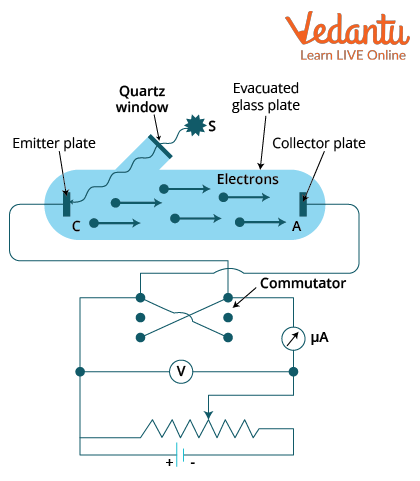Experimental Setup of Photoelectric Effect

Voltage source connected to the emitter and collector is variable and can be changed. Voltmeter measures the voltage, while an ammeter is used to measure the current produced due to the flow of electrons.

## Uses of Photoelectric Effect in Real Life

• Useful for the production of electricity in solar panels consisting of metal fusions that permit electricity production from a huge variety of wavelengths.

• In motion and position sensors, a photoelectric substance is placed inside the light emitting diode (LED). When an object is kept in between the LED and sensor, the light is disconnected and the electronic circuit receives a signal for a change in the potential difference.

• Digital cameras such as digital single-lens reflex (DSLRs) have photoelectric sensors that respond to various colours of light.

• X-Ray photoelectron spectroscopy (XPS) is a methodology that uses x-rays to irradiate a surface and compute the kinetic energies of the discharged electrons. Significant aspects of chemistry, such as the empirical formula of compounds, chemical composition, chemical state, and elemental composition, can be acquired.

• Useful in photomultipliers to capture low levels of light.

• The photoelectric effect is applicable to the night vision devices.

## Conclusion

In general, the photoelectric effect is the emission of electrons when light is incident on metallic objects. It is broadly classified into two types: the internal photoelectric effect and the external photoelectric effect. The major components of this effect are Planck’s equation, threshold energy, and photoelectric equation. Applications of the photoelectric effect are solar panels, cameras, LEDs, night vision appliances, the nuclear industry, and smartphones.

## FAQs on Photoelectric Effect - Important Concepts for JEE

1. What are the necessary conditions for the emission of photoelectrons?

In the photoelectric effect, emission of photoelectrons is only possible from a metallic object. Other than that, there are some necessary conditions to be followed for successful emission of photoelectrons mentioned as follows:

• Energy of incident photons is equal to or greater than the work function of  the metal.

• Frequency of the photons should be above threshold frequency, above which only emission of the photoelectron is possible.

• Energy of the photons should be greater than the energy of the electrons in a metal.

2. What is the major difference between the internal and external photoelectric effect?

Photoelectric effect is defined as the emission of electrons from the surface of metallic objects when incident light fulfilling certain conditions strikes on the surface of the object. The major difference between them is that the internal photoelectric effect causes the production of free electrons as the bounded electrons absorb energy and become free. While in the external photoelectric effect, generation of photocurrent is observed due to the flow of free electrons. Collectively, both effects result in the emission of photoelectrons when light is incident on metallic objects.